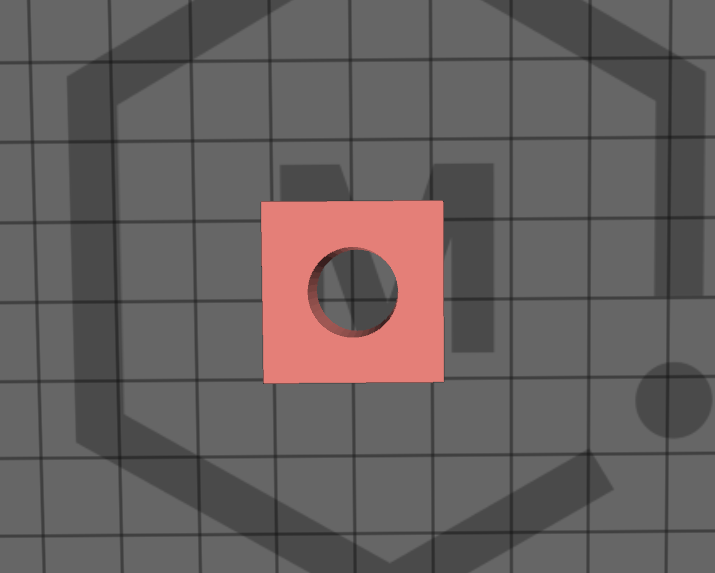# MatterControl Subtract

The way to subtract from an item would be as follows:

1. Load the item you want to subtract something from. Example: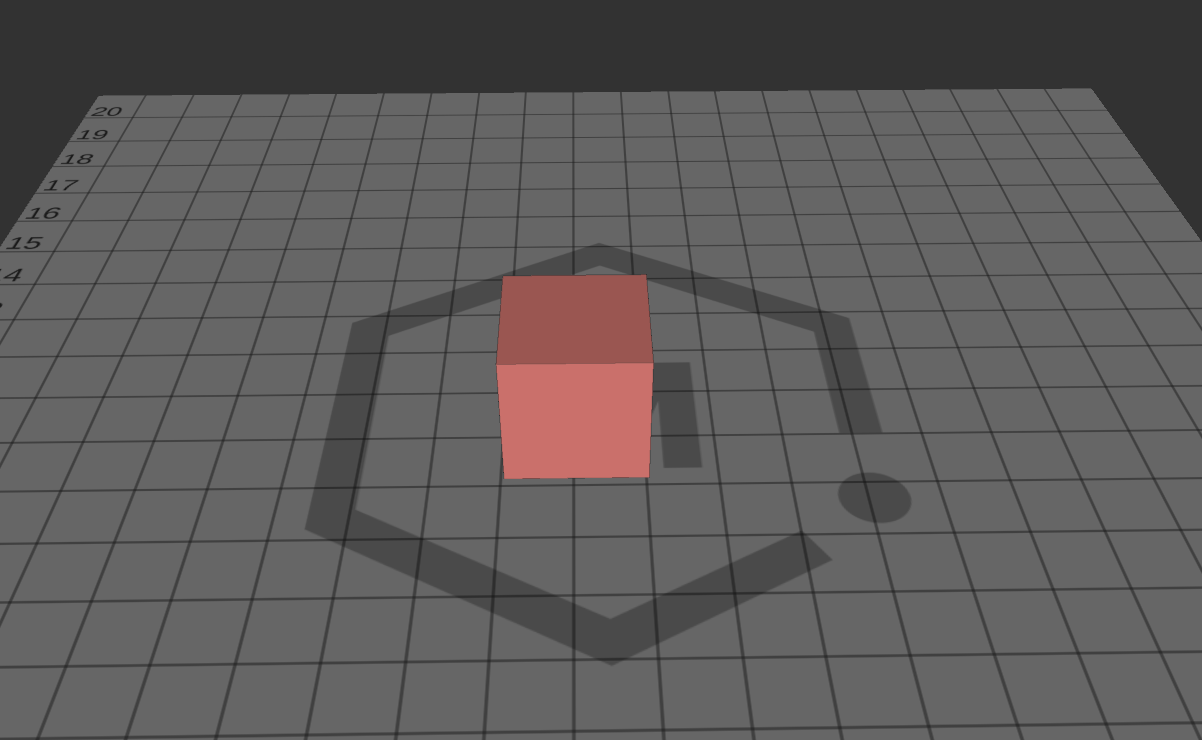2. Add and size another shape in the size you want to subtract from.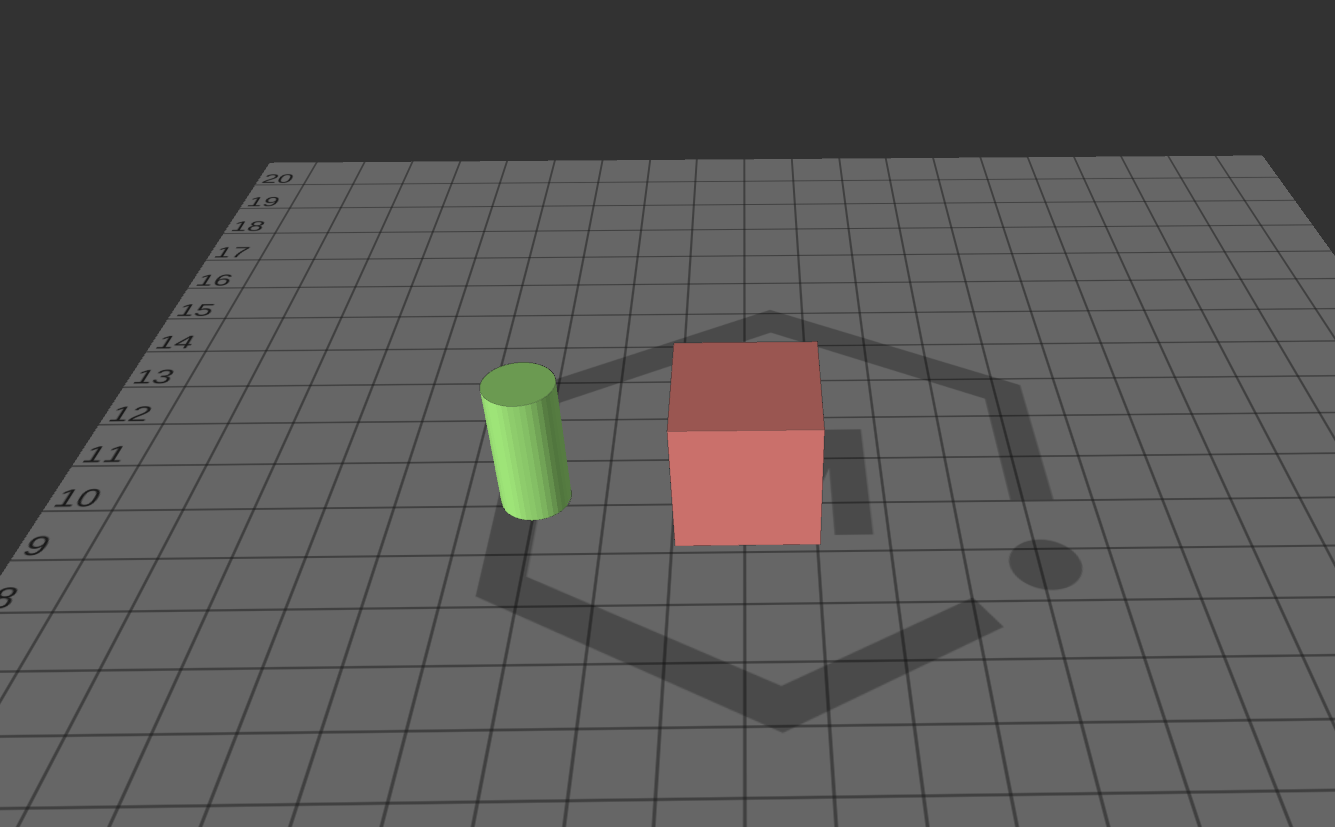3. Position the second shape inside the first in the area you would like to make the subtraction.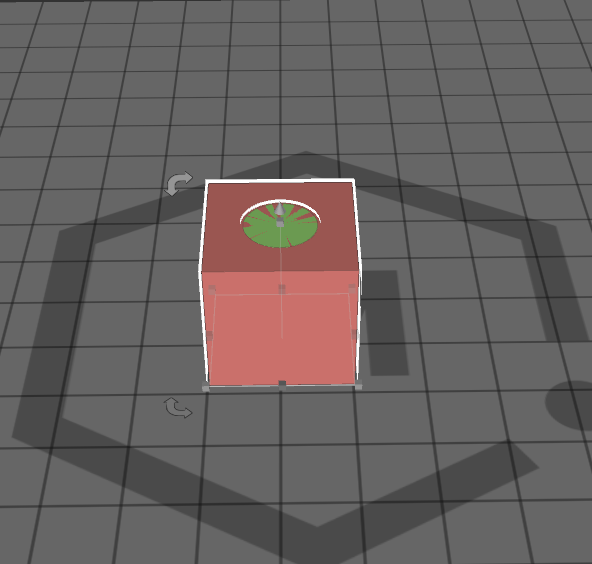4. Select both of the items on your screen and then press the “Subtract” button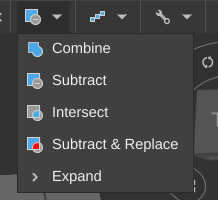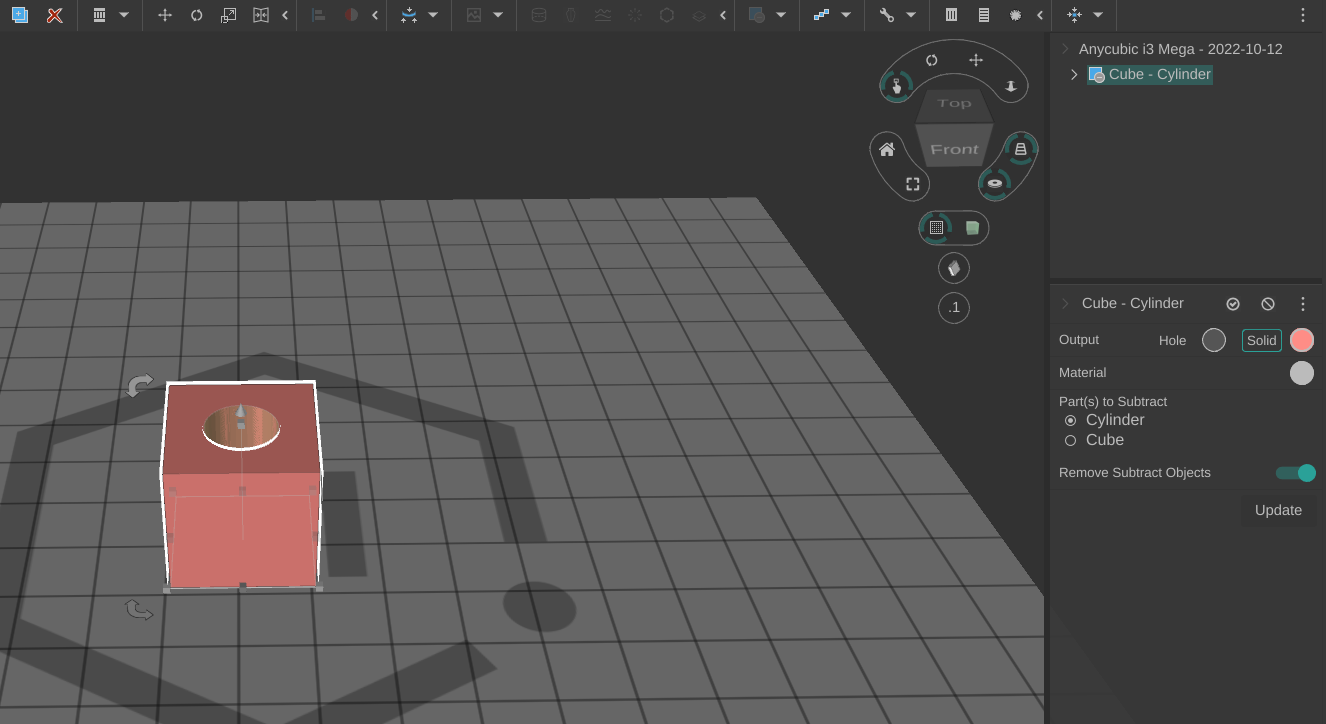5. On the right-hand side, it will ask you which shape will be merged into the other.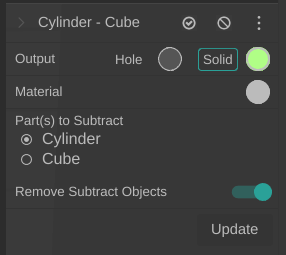6. Hit Update and then it will subtract the item from the other.